With the stock up 20.3% over the prior month, investors may be tempted to sell their shares of Ciena Corporation (NYSE: CIEN). In this article, I am going to calculate the fair value of Ciena by forecasting its future cash flows and discounting them back to today’s value. Value investors may find the results from my analysis surprising.

##### DCF Methodology

The basic philosophy behind a DCF analysis is that the intrinsic value of a company is equal to the future cash flows of that company, discounted back to present value. The general formula is provided below. The intrinsic value is considered the actual value or “true value” of an asset based on an individual’s underlying expectations and assumptions.Cash flows into the firm in the form of revenue as the company sells its products and services, and cash flows out as it pays its cash operating expenses such as salaries or taxes (taxes are part of the definition for cash operating expenses for purposes of defining free cash flow, even though taxes aren’t generally considered a part of operating income). With the leftover cash, the firm will make short-term net investments in working capital (an example would be inventory and receivables) and longer-term investments in property, plant and equipment. The cash that remains is available to pay out to the firm’s investors: bondholders and common shareholders.

I will take you through my own expectations for Ciena as well as explain how I arrived at certain assumptions. The full analysis was completed on Wednesday, March 14. An updated analysis using real-time data can be viewed in your web browser at finbox.io’s Ciena DCF analysis page. The steps involved in the valuation are:

##### `1. Forecast Free Cash Flows`
• Create a revenue forecast
• Forecast EBITDA profit margin
• Calculate free cash flow

##### Step 1: Forecast Free Cash Flows

The key assumptions that have the greatest impact on cash flow projections are typically related to growth, profit margin and investments in the business. The analysis starts at the top of the income statement by creating a forecast for revenue and then works its way down to net operating profit after tax (NOPAT), as shown below.From NOPAT, deduct cash outflows like capital expenditures and investments in net working capital and add back non-cash expenses from the income statement such as depreciation and amortization to calculate the unlevered free cash flow forecast (shown above).

###### Create A Revenue Forecast

When available, the finbox.io’s pre-built models use analyst forecasts as the starting assumptions. To forecast revenue, analysts gather data about the company, its customers and the state of the industry. I typically review the analysts’ forecast and modify the growth rates based on historical performance, news and other insights gathered from competitors. Note that if a company only has a small number of analysts giving projections, the consensus forecast tends to not be as reliable as companies that have several analysts’ estimates. Another check for reliability is to analyze the range of estimates. If the range is really wide, it may be less accurate.

Ciena provides hardware, software, and services that support the transport, service, and management of voice, video, and data traffic on communications networks worldwide. The company was founded in 1992 and is headquartered in Hanover, Maryland. Analysts covering the stock often compare the company to a peer group that includes Juniper Networks, Inc. (NYSE: JNPR), Arista Networks, Inc. (NYSE: ANET), Motorola Solutions, Inc. (NYSE: MSI), and ARRIS International plc (NASDAQ: ARRS).

The company’s 5-year revenue CAGR of 8.8% is above its selected comparable public companies: JNPR (2.9%) and MSI (0.4%) but below ANET (53.5%) and ARRS (37.3%).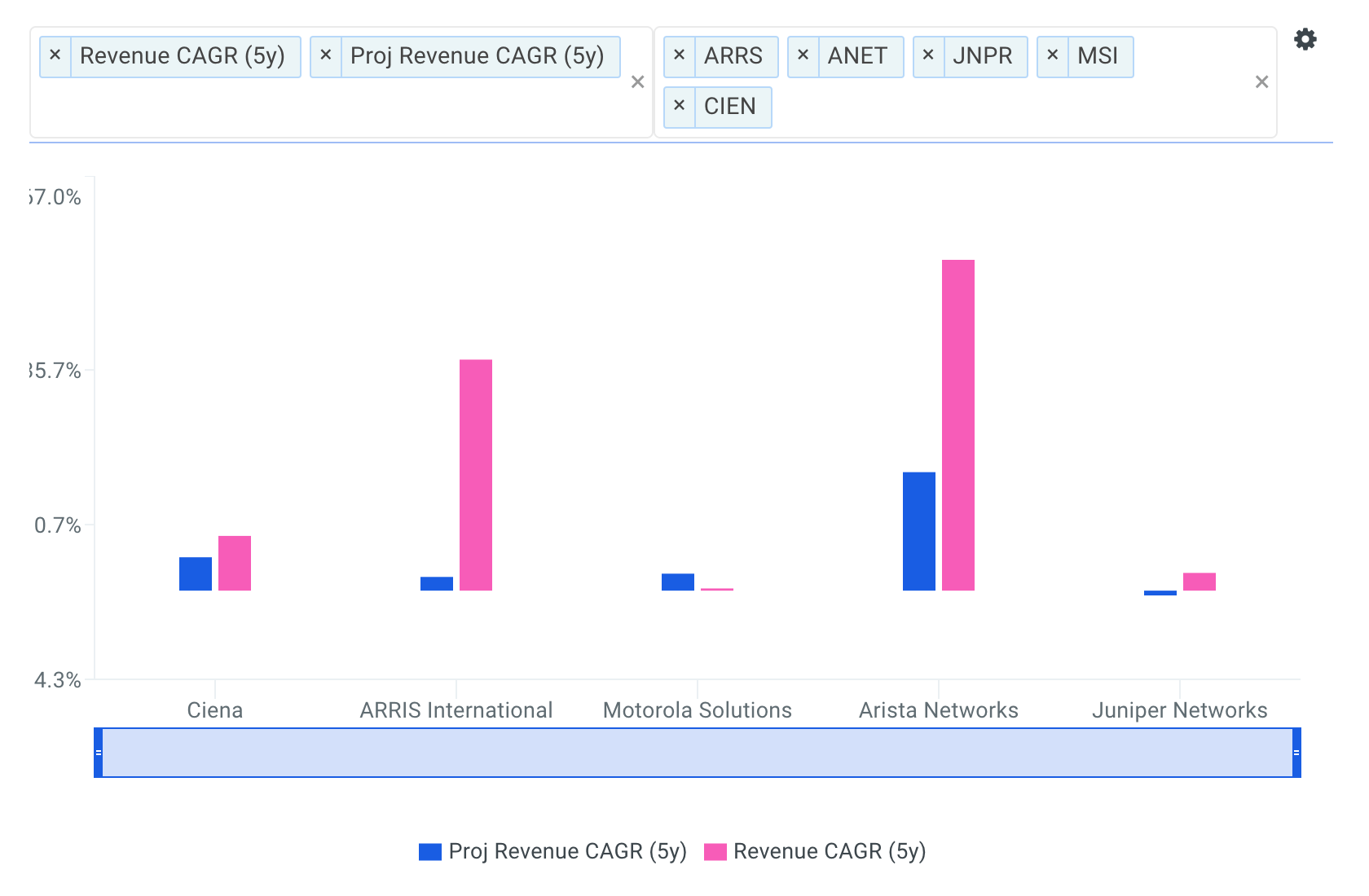source: Benchmarks: Forecasted 5yr Revenue CAGRs

The company’s projected 5-year revenue CAGR of 5.4% is above JNPR (-0.8%), MSI (2.7%) and ARRS (2.2%) and only below ANET (19.1%).

As highlighted below, Ciena’s revenue growth has ranged from 6.3% to 13.6% over the last five fiscal years.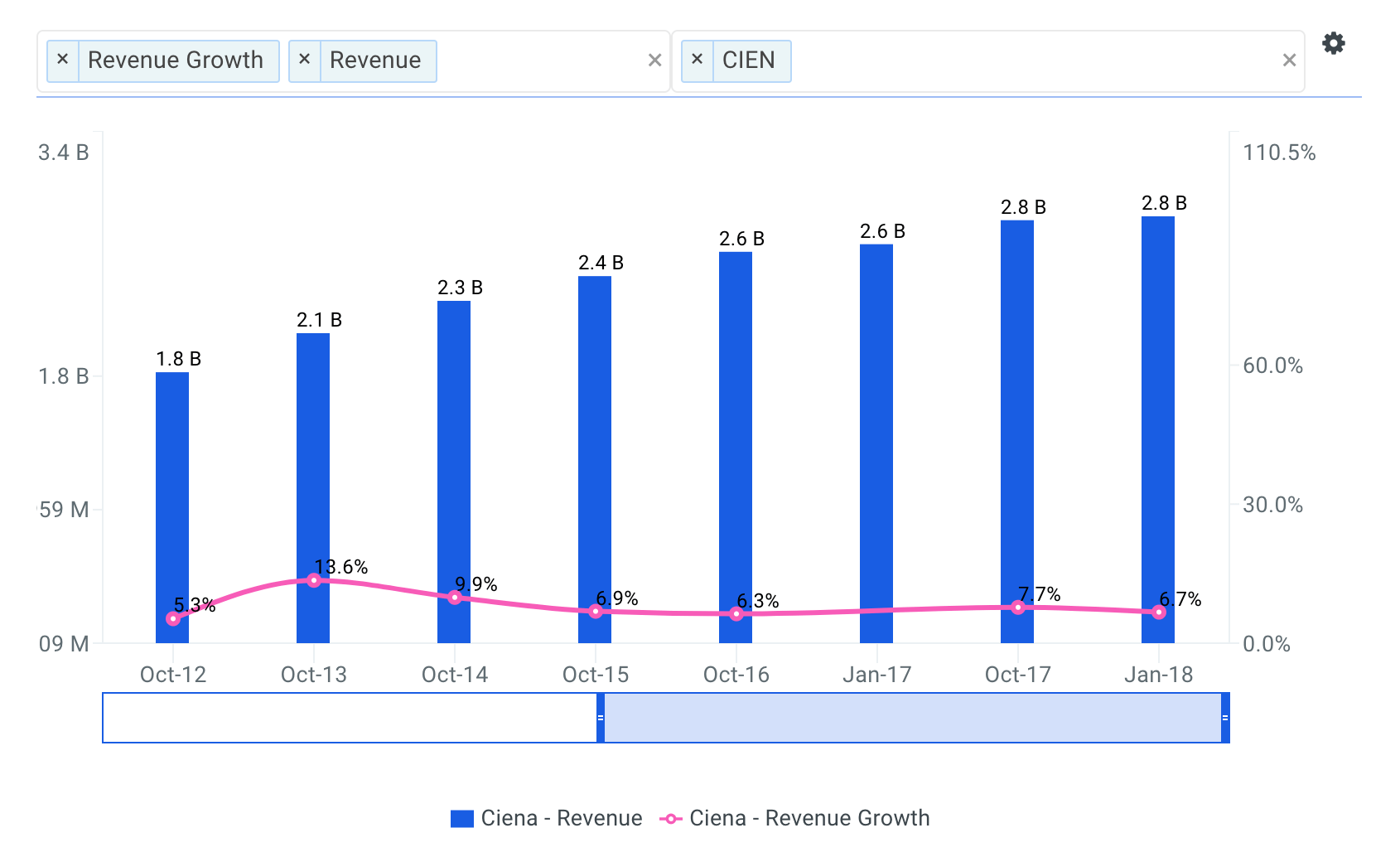Going forward, analysts forecast that Ciena’s total revenue will reach \$3,643 million by fiscal year 2022 representing a five-year CAGR of 5.4%.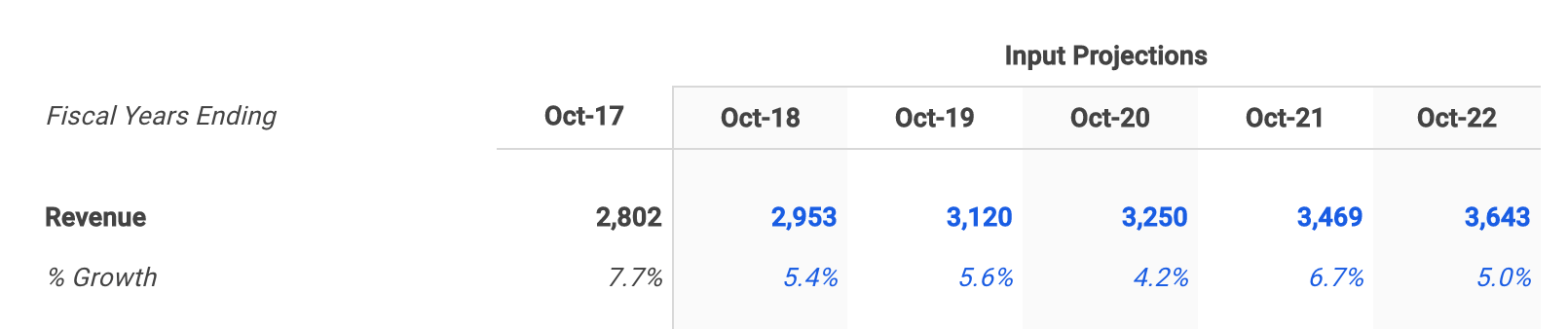###### Forecast Ciena’s EBITDA Profit Margin

The next step is to forecast the company’s earnings before interest, taxes, depreciation and amortization (EBITDA). Note that EBITDA is a commonly used metric in valuation models because it provides a cleaner picture of overall profitability, especially when benchmarking against comparable companies. This is because it ignores non-operating costs that can be affected by certain items such as a company’s financing decisions or political jurisdictions. For more detail, see Ciena’s EBITDA definition.

EBITDA margin is calculated by dividing EBITDA by revenue. The higher the EBITDA margin, the smaller the firm’s operating expenses are in relation to its revenue, which may ultimately lead to higher profit. Lower operating expenses for a given level of revenue can be a sign of internal economies of scale.

The charts below compare Ciena’s LTM EBITDA margin to the same peer group. The company’s EBITDA margin of 11.5% is only above ARRS (9.9%) and below JNPR (21.4%), ANET (29.9%) and MSI (25.5%).

Ciena’s EBITDA margin has ranged from 6.0% to 12.1% over the last five fiscal years.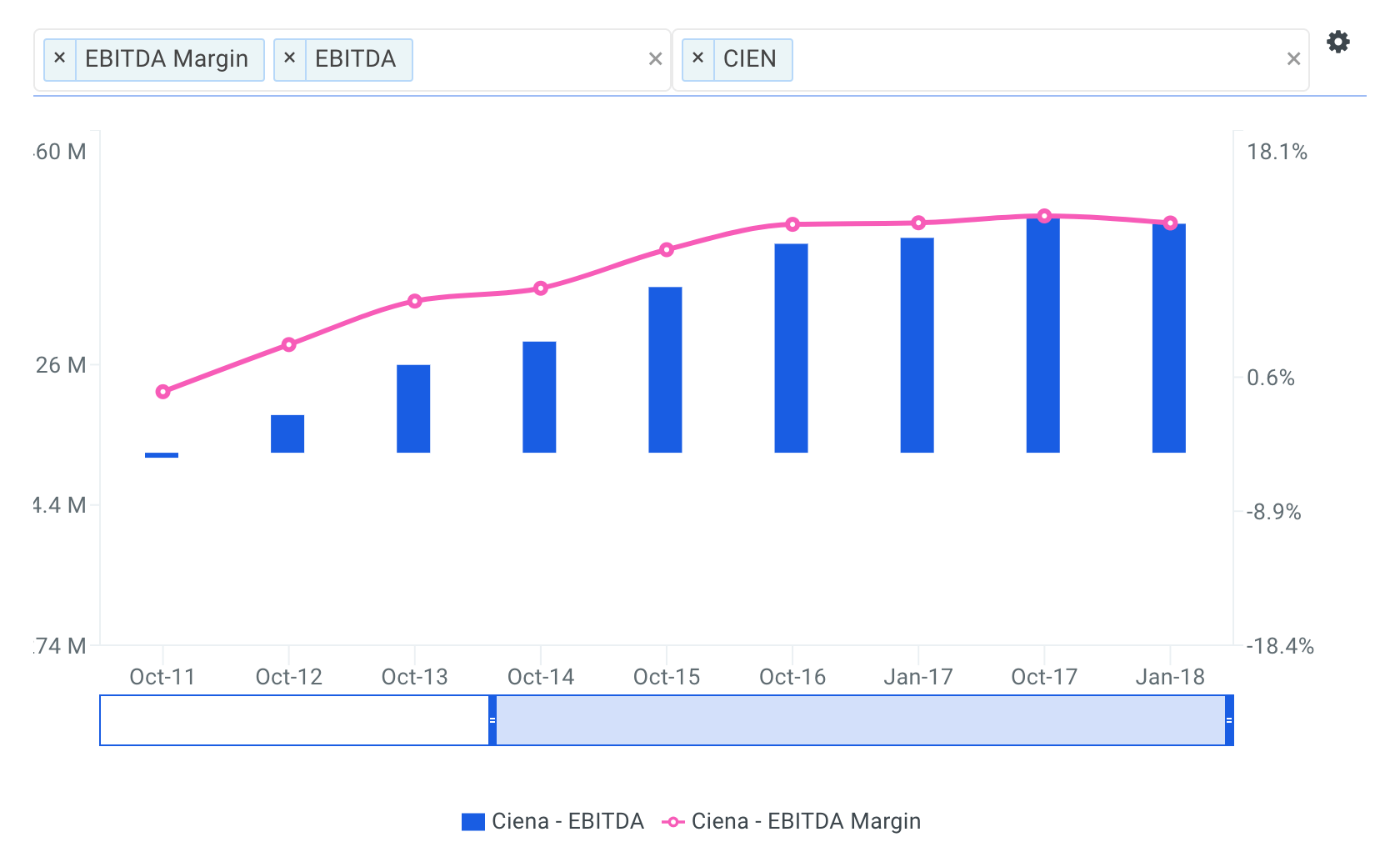Wall Street analysts are forecasting that Ciena’s EBITDA margin will reach 17.8% by fiscal year 2022, representing an increase of 6.2% from its LTM EBITDA margin of 11.5%.Although I don’t specifically walk through my assumptions here, I then forecasted depreciation & amortization, capital expenditures and net working capital based on historical levels.

###### Calculate Free Cash Flow

With all required forecasts in place, the next step is to calculate projected free cash flow as shown below.##### Step 2: Select Ciena’s Discount Rate

The next step is to select a discount rate to calculate the present value of the forecasted free cash flows. I used finbox.io’s Weighted Average Cost of Capital (WACC) model to help arrive at an estimate. Generally, a company’s assets are financed by either debt (debt is after tax in the formula) or equity. WACC is the average return expected by these capital providers, each weighted by respective usage. The WACC is the required return on the firm’s assets.

It’s important to note that the WACC is the appropriate discount rate to use because this analysis calculates the free cash flow available to Ciena’s bondholders and common shareholders. On the other hand, the cost of equity would be the appropriate discount rate if we were calculating cash flows available only to Ciena’s common shareholders (i.e., dividend discount model, equity DCF). This is commonly referred to as the difference between free cash flow to equity (FCFE) and free cash flow to the firm (FCFF). By using the WACC to discount FCFF, we are calculating total firm value. If we discounted FCFE at the required return on equity, we would end up with equity value of the firm. Equity value of the firm is simply total firm value minus the market value of debt.

I determined a reasonable WACC estimate for Ciena to be 10.5% at the midpoint. An updated cost of capital analysis using real-time data can be found at finbox.io’s Ciena WACC Model Page. The DCF model then does the heavy lifting of calculating the discount factors by applying the mid-year convention technique.

##### Step 3: Estimate Ciena’s Terminal Value

Since it is not reasonable to expect that Ciena will cease its operations at the end of the five-year forecast period, we must estimate the company’s continuing value, or terminal value. Terminal value is an important part of the DCF model because it accounts for the largest percentage of the calculated present value of the firm. If you were to exclude the terminal value, you would be excluding all the future cash flow past the horizon period. Using finbox.io, users can choose a five-year or 10-year horizon period to forecast future free cash flow.

The most generally accepted techniques to calculate a terminal value are by applying the Gordon growth approach, using an EBITDA exit multiple and using a revenue exit multiple. This analysis applies the Gordon growth formula: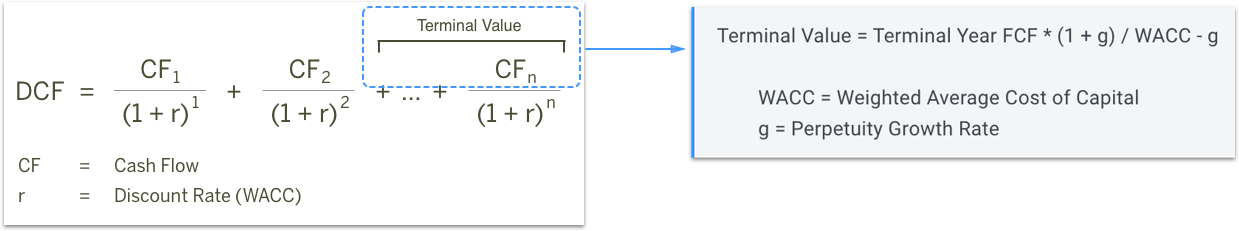As the formula suggests, we need to estimate a “perpetuity” growth rate at which we expect Ciena’s free cash flows to grow forever. Most analysts suggest that a reasonable rate is typically between the historical inflation rate of 2% to 3% and the historical GDP growth rate of 4% to 5%.

I’ve selected a conservative perpetuity growth rate of 3.0% (at the midpoint) even though Ciena’s free cash flows are still growing well above that at the end of the projection period.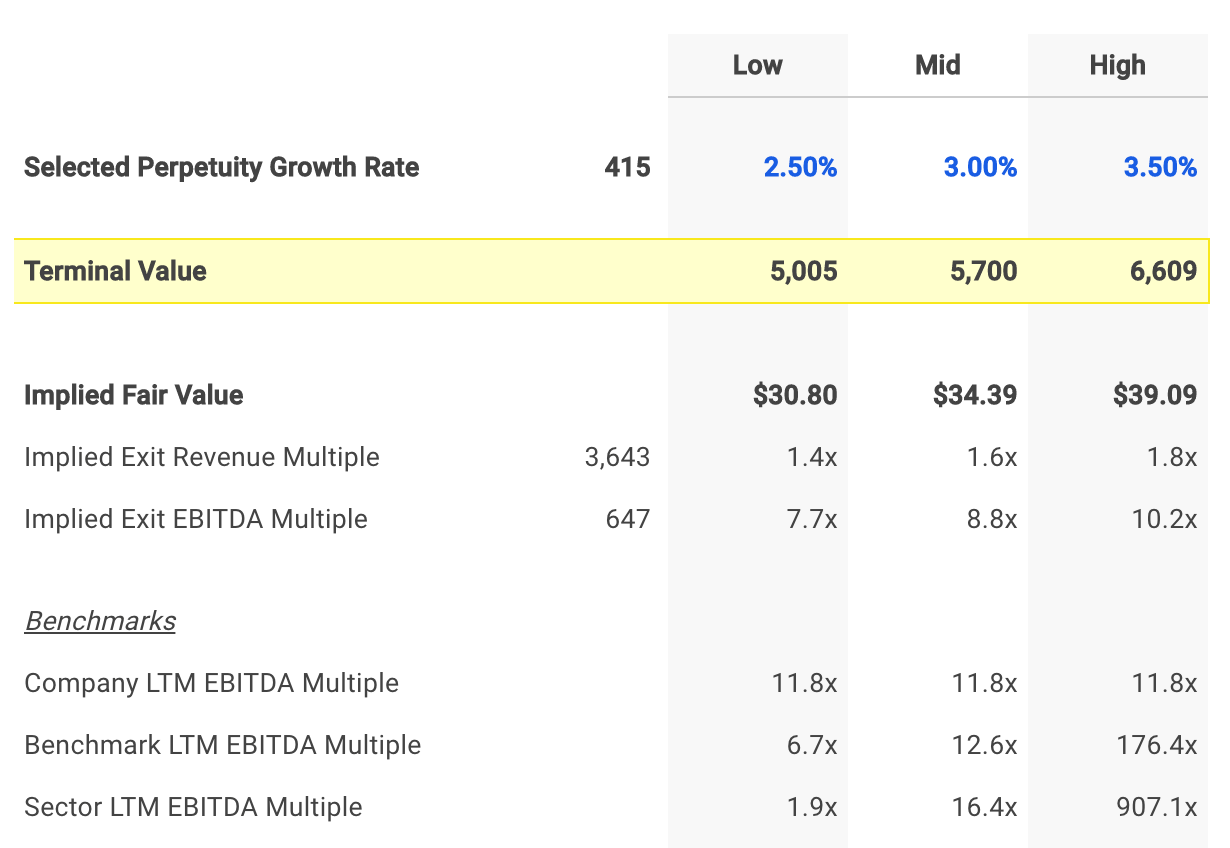An EBITDA multiple is calculated by dividing enterprise value by EBITDA. Similarly, the terminal EBITDA multiple implied from a DCF analysis is calculated by dividing the terminal value by the terminal year’s projected EBITDA. Comparing the terminal EBITDA multiple implied from the selected growth rate to benchmark multiples can serve as a useful check.Ciena’s implied exit EBITDA multiple of 8.8x is at the low end of where its peers are trading. This means my selected perpetuity growth rate of 3.0% is likely conservative.

##### Step 4: Calculate Ciena’s Equity Value

The enterprise value previously calculated is a measure of the company’s total value. An equity waterfall is a term often used by valuation firms, referring to the trickle-down process of computing a company’s equity value from its enterprise value. Note that in the event of a bankruptcy, debt holders will be paid in full before anything is distributed to common shareholders. Therefore, we must subtract debt and other financial obligations to determine a firm’s equity value. The general formula for calculating equity value is illustrated in the figure below.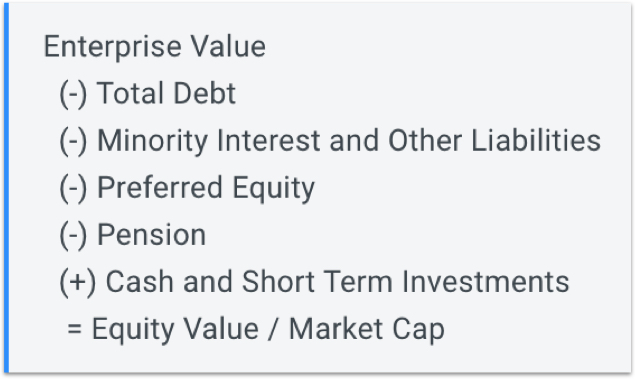The model uses the formula shown above to calculate equity value and divides the result by the shares outstanding to compute intrinsic value per share as shown at the bottom of the figure below.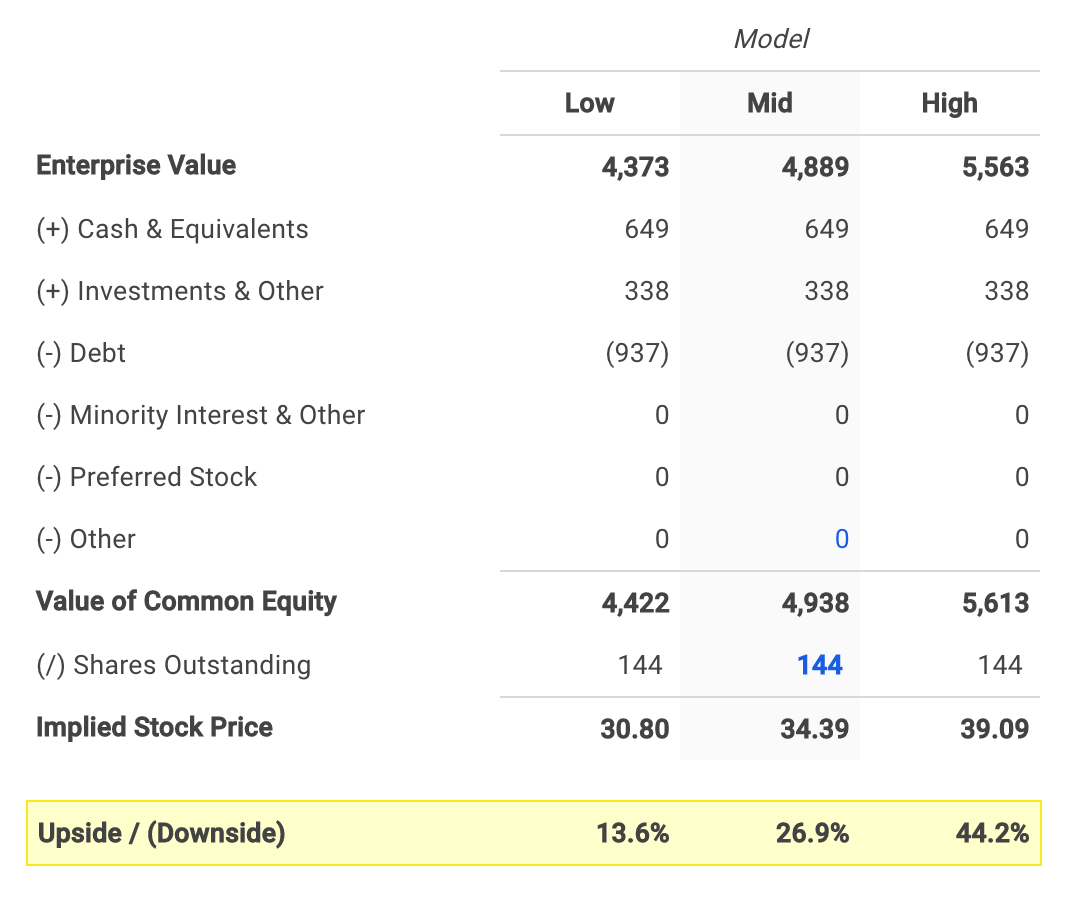The assumptions I used in the model imply an intrinsic value per share range of \$30.80 to \$39.09 for Ciena.

Ciena’s stock price currently trades at \$26.94 as of Wednesday, March 14th, 26.9% below the midpoint value of \$34.39.

##### Conclusion: Ciena Still Has Upside Potential

A DCF analysis can seem complex at first, but it’s worth adding to your investment analysis toolbox since it provides the clearest view of company value.

Ciena’s stock has made impressive gains over the prior month and investors may very well decide to sell their shares. However, the stock still appears to have further upside potential based on its future cash flow projections.

A DCF analysis is only one side of the coin in terms of building your investment thesis, and it shouldn’t be the only tool you use when researching a company. I recommend you continue to research Ciena to get a more comprehensive view of the company by looking at:

Risk Metrics: what is Ciena’s asset efficiency? This ratio measures the amount of cash flow that a company generates from its assets. View the company’s asset efficiency here.

Efficiency Metrics: fixed asset turnover is calculated by dividing revenue by average fixed assets. View Ciena’s fixed asset turnover here.

Forecast Metrics: what is Ciena’s projected earnings growth? Is the company expected to grow faster or slower relative to its peers? Analyze the company’s projected earnings growth here.

##### Author: Matt Hogan

Expertise: Valuation, financial statement analysis

Matt Hogan is also a co-founder of finbox.io. His expertise is in investment decision making. Prior to finbox.io, Matt worked for an investment banking group providing fairness opinions in connection to stock acquisitions. He spent much of his time building valuation models to help clients determine an asset’s fair value. He believes that these same valuation models should be used by all investors before buying or selling a stock.

His work is frequently published at InvestorPlace, Benzinga, ValueWalk, AAII, Barron’s, Seeking Alpha and investing.com.

Matt can be reached at matt@finbox.io.

This is not a buy or sell recommendation on any security mentioned.
image source

Read more awesome articles like this one on VintageValueInvesting.com!